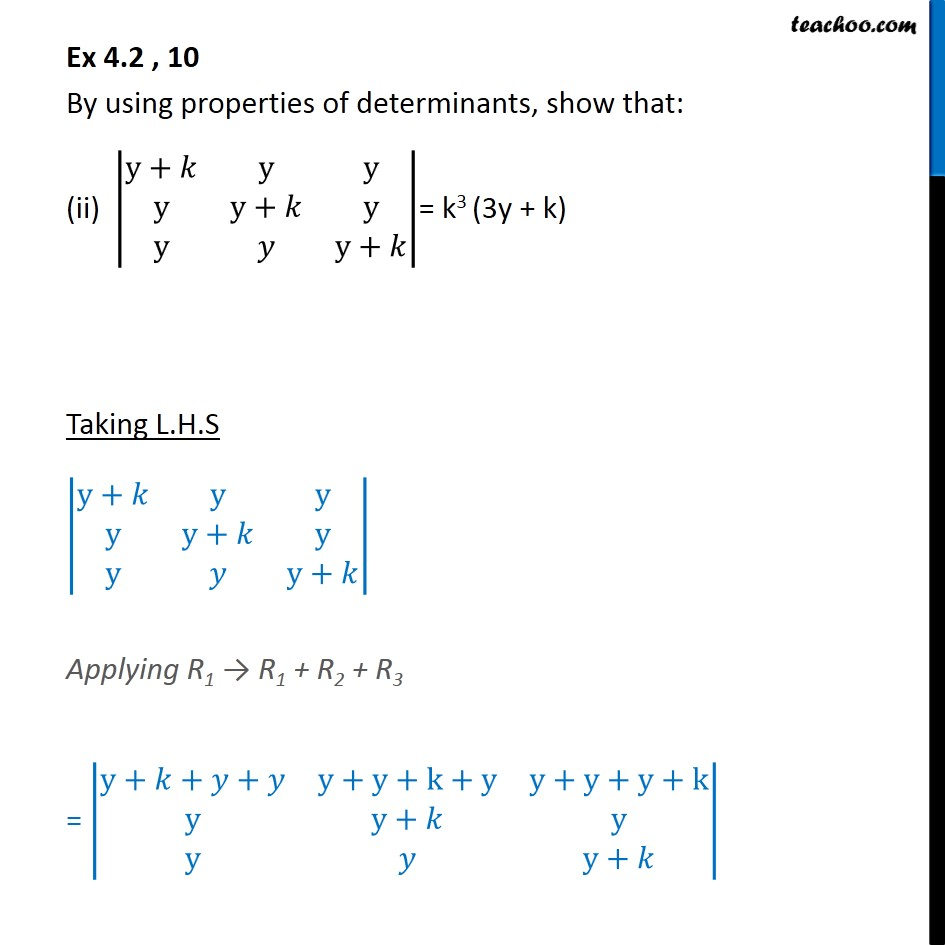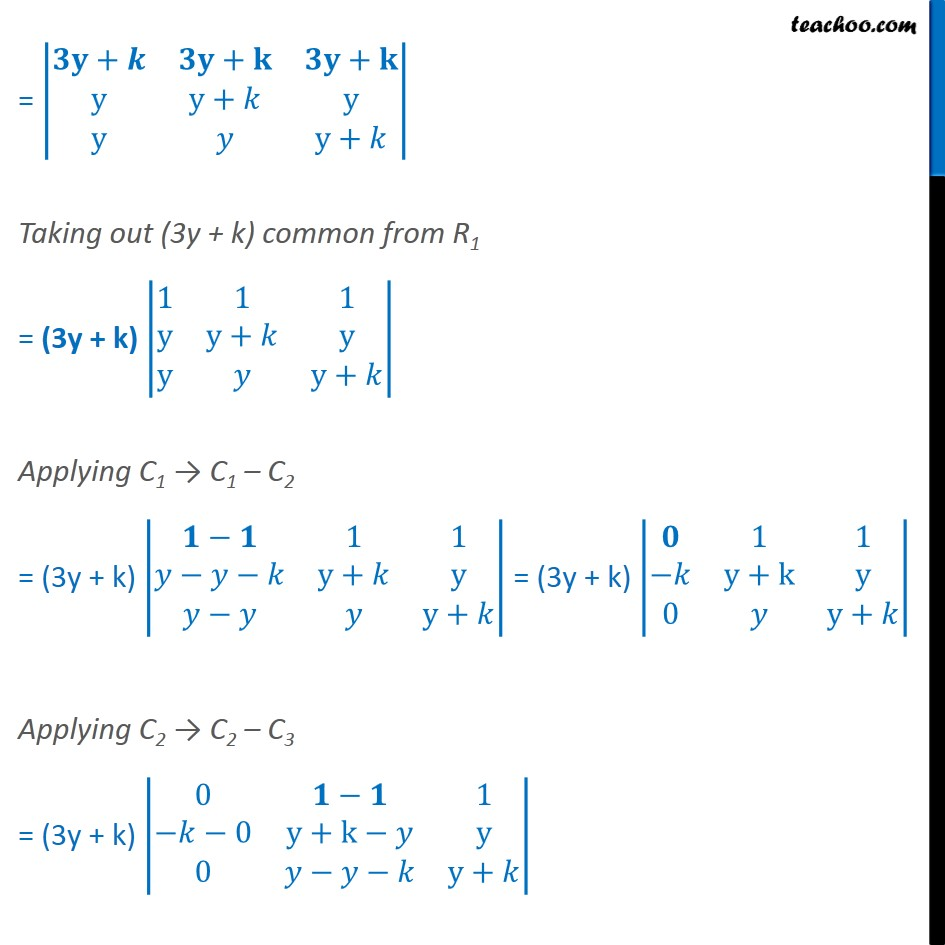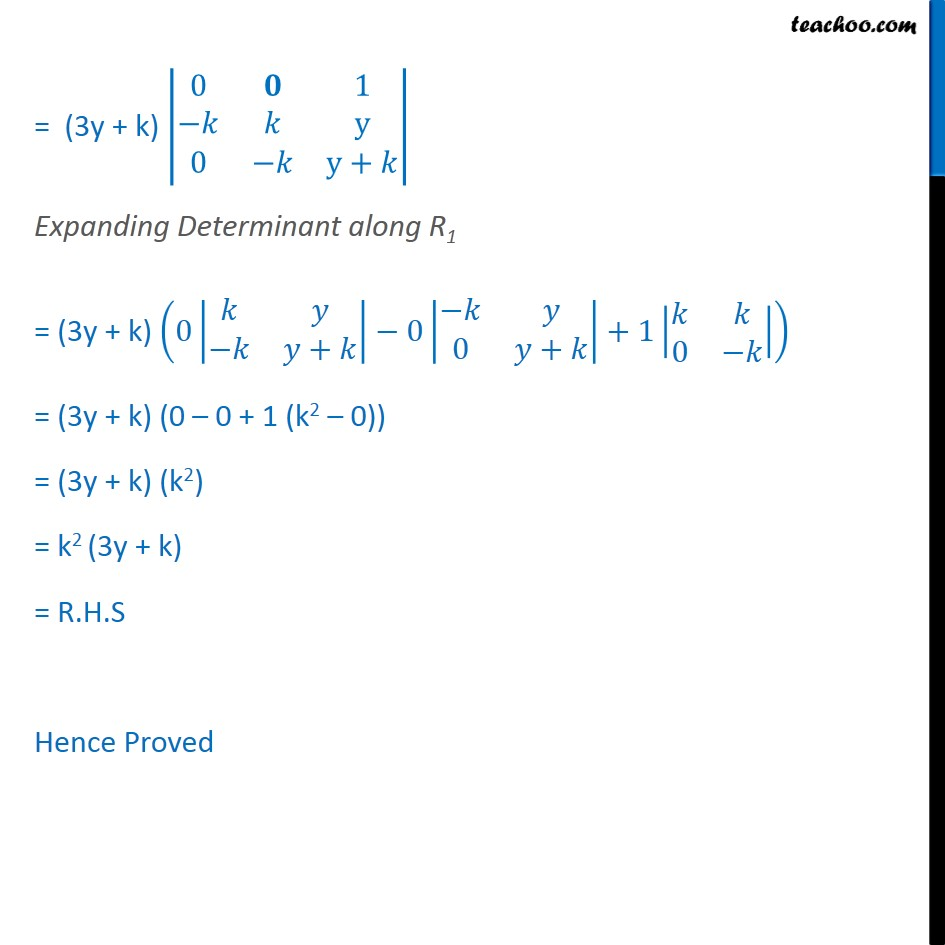Properties of Determinant

Chapter 4 Class 12 Determinants
Serial order wiseLearn in your speed, with individual attention - Teachoo Maths 1-on-1 Class

### Transcript

Ex 4.2 , 10 By using properties of determinants, show that: (ii) y+ y y y y+ y y y+ = k3 (3y + k) Taking L.H.S y+ y y y y+ y y y+ Applying R1 R1 + R2 + R3 = y+ + + y+y+k+y y+y+y+k y y+ y y y+ = + + + y y+ y y y+ Taking out (3y + k) common from R1 = (3y + k) 1 1 1 y y+ y y y+ Applying C1 C1 C2 = (3y + k) 1 1 y+ y y+ = (3y + k) 1 1 y+k y 0 y+ Applying C2 C2 C3 = (3y + k) 0 1 0 y+k y 0 y+ = (3y + k) 0 1 y 0 y+ Expanding Determinant along R1 = (3y + k) 0 + 0 0 + +1 0 = (3y + k) (0 0 + 1 (k2 0)) = (3y + k) (k2) = k2 (3y + k) = R.H.S Hence Proved